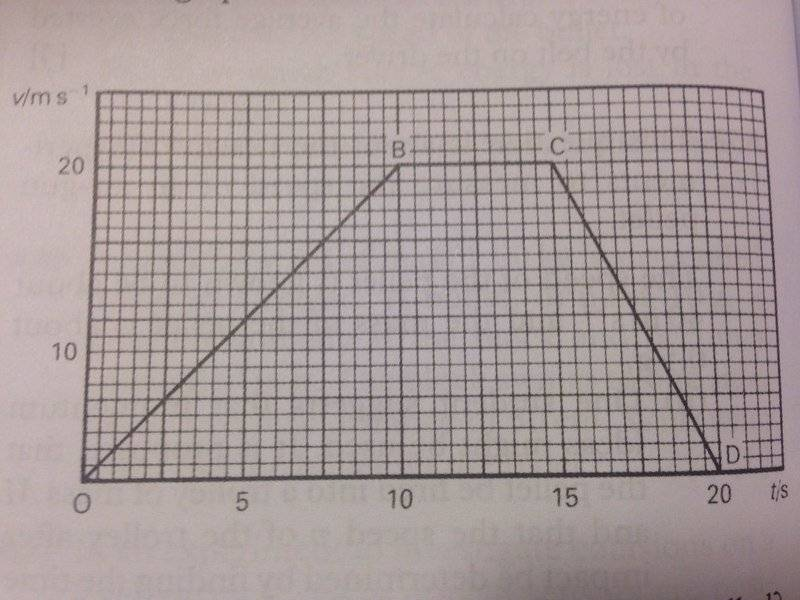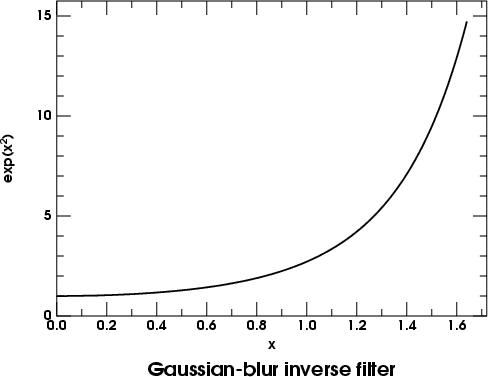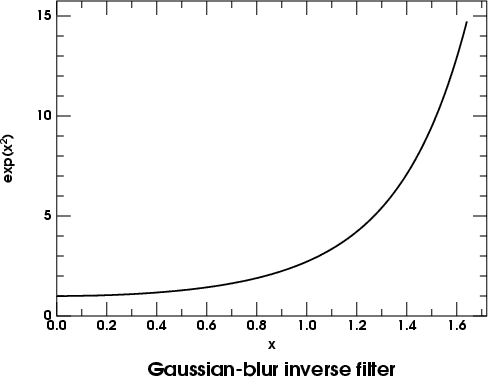# Driving force over time to produce acceleration (graph)

## Homework Statement

Parts which I am not sure about are in bold.

(a) A car of mass 1000 kg is initially at rest. It moves along a straight road for 20 s and then comes to rest again. The speed-time graph for the movement is:(i) What is the total distance travelled?
(ii) What resultant force acts on the car during the part of the motion represented by CD?
(iii) What is the momentum of the car when it has reached its maximum speed? Use this momentum value to find the constant resultant accelerating force.
(iv) During the part of the motion represented by OB on the graph, the constant resultant force found in (iii) is acting on the moving car although it is moving through air. Sketch a graph to show how the driving force would have to vary with time to produce this constant acceleration. Explain the shape of your graph.

(b) If, when travelling at this maximum speed, the 1000 kg car had struck and remained attached to a stationary vehicle of mass 1500 kg, with what speed would the interlocked vehicles have travelled immediately after collision?

Calculate the kinetic energy of the car just prior to this collision and the kinetic energy of the interlocked vehicles just afterwards. Common upon the values obtained.

Explain how certain design features in a modern car help to protect the driver of a car in such a collision.

2. The attempt at a solution
(a) (i) Total distance = 250 m.
(ii) F = m * (v / t) = 1000 * (20 / 5) = 4000 N
(iii) p = mv = 1000 * 20 = 20 000 kg / ms
F = p / t = 20 000 / 10 = 2000 N
(iv) Shouldn't the graph be a linear graph just a horizontal line? On the given graph we have velocity / time and that means that the acceleration is constant (velocity is increasing). Because of constant velocity, the driving force F = ma is constant as well (m and a don't change). So in that case I would draw the graph a horizontal line. Is this logic right?

(b) 20 * 1000 = v (1000 + 1500) -> v = 8 m / s
KE = 1/2 mv2 = 0.5 * 1000 * 202 = 200 000 J
KE = 0.5 * 2500 * 82 = 80 000 J

The car had 200 kJ before and the combined KE is 80 kJ. So we can conclude that there was a serious accident and 120 kJ have transformed into sound and head.

Seatbelts, airbags, bumpers? Or the whole car is made out of a better material so when there is an collision the car doesn't deform much and the KE doesn't transform into heat and sound?

haruspex
Homework Helper
Gold Member
2020 Award
For a iv), they want you to consider drag. But they have not told you how drag depends on speed, which is unusual. If you have not been taught anything about that, assume it is quadratic.

For the last part, you want a car to deform in a serious collision. That's how it absorbs the energy of the impact. But you don't want the passenger compartment to collapse, so cars have front and rear crumple zones. These are designed to provide a more-or-less constant resistance as they are crushed. That minimises the maximum forces on the passengers.

•moenste
collinsmark
Homework Helper
Gold Member

## Homework Statement

Parts which I am not sure about are in bold.

(a) A car of mass 1000 kg is initially at rest. It moves along a straight road for 20 s and then comes to rest again. The speed-time graph for the movement is:(i) What is the total distance travelled?
(ii) What resultant force acts on the car during the part of the motion represented by CD?
(iii) What is the momentum of the car when it has reached its maximum speed? Use this momentum value to find the constant resultant accelerating force.
(iv) During the part of the motion represented by OB on the graph, the constant resultant force found in (iii) is acting on the moving car although it is moving through air. Sketch a graph to show how the driving force would have to vary with time to produce this constant acceleration. Explain the shape of your graph.

(b) If, when travelling at this maximum speed, the 1000 kg car had struck and remained attached to a stationary vehicle of mass 1500 kg, with what speed would the interlocked vehicles have travelled immediately after collision?

Calculate the kinetic energy of the car just prior to this collision and the kinetic energy of the interlocked vehicles just afterwards. Common upon the values obtained.

Explain how certain design features in a modern car help to protect the driver of a car in such a collision.

2. The attempt at a solution
(a) (i) Total distance = 250 m.
(ii) F = m * (v / t) = 1000 * (20 / 5) = 4000 N
(iii) p = mv = 1000 * 20 = 20 000 kg / ms

Check your units on part (iii). Otherwise, so far so good.

F = p / t = 20 000 / 10 = 2000 N
(iv) Shouldn't the graph be a linear graph just a horizontal line? On the given graph we have velocity / time and that means that the acceleration is constant (velocity is increasing). Because of constant velocity, the driving force F = ma is constant as well (m and a don't change). So in that case I would draw the graph a horizontal line. Is this logic right?
I think it's asking you to account for air resistance. The "resultant force" is constant, and you have calculated that. What it's saying is that the "driving force" is not necessarily constant, and might vary to account for air resistance in order to keep the "resultant force" constant.

(b) 20 * 1000 = v (1000 + 1500) -> v = 8 m / s
KE = 1/2 mv2 = 0.5 * 1000 * 202 = 200 000 J
KE = 0.5 * 2500 * 82 = 80 000 J

The car had 200 kJ before and the combined KE is 80 kJ. So we can conclude that there was a serious accident and 120 kJ have transformed into sound and head.

Seatbelts, airbags, bumpers?
Those are all good answers.Or the whole car is made out of a better material so when there is an collision the car doesn't deform much and the KE doesn't transform into heat and sound?

You might want to rethink that part though. The goal is to keep the driver and passengers from deforming (by keeping their acceleration to a minimum). It's not so bad if other parts of the car deform though.

•moenste
For a iv), they want you to consider drag. But they have not told you how drag depends on speed, which is unusual. If you have not been taught anything about that, assume it is quadratic.
I think it's asking you to account for air resistance. The "resultant force" is constant, and you have calculated that. What it's saying is that the "driving force" is not necessarily constant, and might vary to account for air resistance in order to keep the "resultant force" constant.
"Although it is moving through air" = so we need to consider air resistance.

But if we have Driving force F - Resistance R = Resultant force 2000 N and we have constant resistance (e.g. constant air resistance) should't the graph still be linear, a horizontal line of F? (if we consider that F, R and 2000 N are always constant). And in case the R is increasing over time then F also increases and the graph should be a line which increases like OB on the given graph. (over time we need more driving force to keep the resultant force the same).

I'm mostly not sure about the graph.

Check your units on part (iii). Otherwise, so far so good.
Yes, it should be kg m / s (kg m s-1).

For the last part, you want a car to deform in a serious collision. That's how it absorbs the energy of the impact. But you don't want the passenger compartment to collapse, so cars have front and rear crumple zones. These are designed to provide a more-or-less constant resistance as they are crushed. That minimises the maximum forces on the passengers.
You might want to rethink that part though. The goal is to keep the driver and passengers from deforming (by keeping their acceleration to a minimum). It's not so bad if other parts of the car deform though.
Hm, I meant that modern cars have good frames (e.g. like in a Smart car), so when there is a collusion these frames keep the car (the passenger part of the car) from completely deforming. But I understand it better after looking at the crumple zones which haruspex said. In regular vehicles the crumple zones are at the front and back of the car and the passenger part is protected with a frame.

So, in sum: in order to protect the passenger(s) in an accident, there are two types of protection. The first type is in the cabin: seatbelts and airbags which are deisgned to keep the passengers' acceleration to a minimum during a crash. And the second type are crumple zones with frames which allow the car to deform at the back and front but due to frames the passenger section remains relatively safe / untouched / unaffected.

---

P. S. [...] 120 kJ have transformed into sound and head -> heat, a typo :).

haruspex
Homework Helper
Gold Member
2020 Award
But if we have Driving force F - Resistance R = Resultant force 2000 N and we have constant resistance (e.g. constant air resistance)
But that's exactly the point, air resistance is not constant. It depends on speed. No speed, no resistance. Typically, it is taken to rise quadratically with speed, but that's just an approximation and breaks down in some regimes.

•moenste
But that's exactly the point, air resistance is not constant. It depends on speed. No speed, no resistance. Typically, it is taken to rise quadratically with speed, but that's just an approximation and breaks down in some regimes.
So what's the correct graph in this situation?

One like this? (X = time, Y = driving force). This shape is due to air resistance which increases (as you say) quadratically with speed.haruspex•